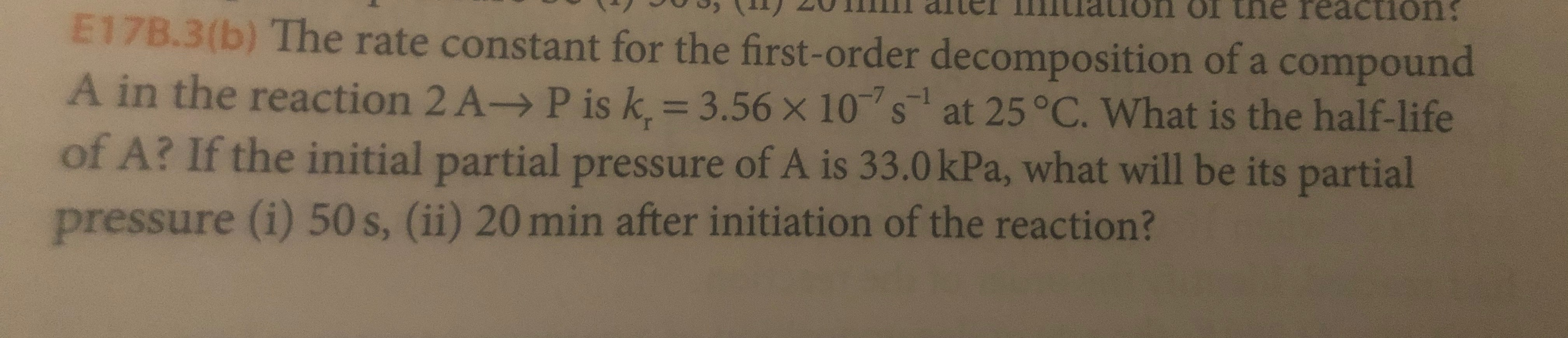# aion ol tne reactionE17B.3(b) The rate constant for the first-order decomposition of a compoundA in the reaction 2 A-> P is k. 3.56 x 10s at 25 °C. What is the half-lifeof A? If the initial partial pressure of A is 33.0 kPa, what will be its partialpressure (i) 50 s, (ii) 20 min after initiation of the reaction?

Question
9 viewshelp_outlineImage Transcriptioncloseaion ol tne reaction E17B.3(b) The rate constant for the first-order decomposition of a compound A in the reaction 2 A-> P is k. 3.56 x 10s at 25 °C. What is the half-life of A? If the initial partial pressure of A is 33.0 kPa, what will be its partial pressure (i) 50 s, (ii) 20 min after initiation of the reaction? fullscreen
check_circle

Step 1

The term first order reaction is defined for those reactions which proceed at a rate that has a linear dependence on the concentration of only one reactant.

Step 2

The half-life of a given reaction can be defined as the time in which the initial concentration of the reactants reduces to half of its original value. Mathematically it can be represented as follows:

Step 3

For the given reaction, the first order rate constant is given to be 3.56×10-7 s-1.

T...

### Want to see the full answer?

See Solution

#### Want to see this answer and more?

Solutions are written by subject experts who are available 24/7. Questions are typically answered within 1 hour.*

See Solution
*Response times may vary by subject and question.
Tagged in

### Physical Chemistry